# IA 32 Floating Point History n Instruction decoder

• Slides: 36IA 32 Floating Point History n Instruction decoder and sequencer 8086: first computer to implement IEEE FP l separate 8087 FPU (floating point unit) n 486: merged FPU and Integer Unit onto one chip Summary n n n Hardware to add, multiply, and divide Floating point data registers Various control & status registers Integer Unit FPU Floating Point Formats n n n – 1– single precision (C float): 32 bits double precision (C double): 64 bits extended precision (C long double): 80 bits Memory CSCI-2500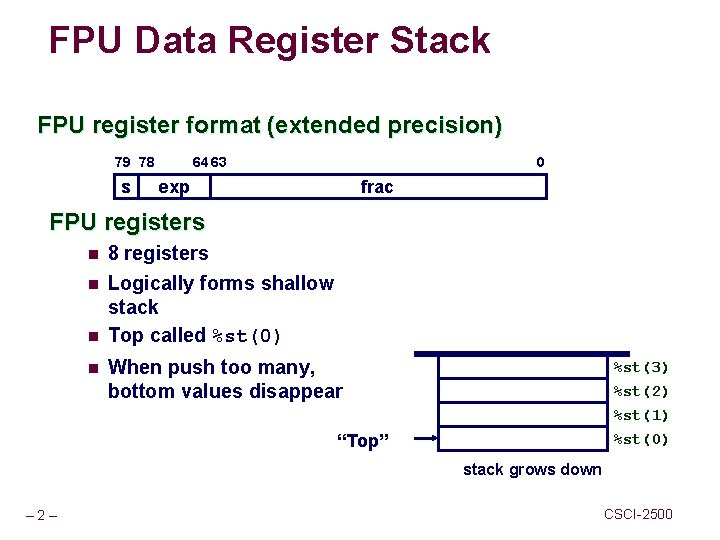FPU Data Register Stack FPU register format (extended precision) 79 78 s 0 64 63 exp frac FPU registers n 8 registers n Logically forms shallow stack Top called %st(0) n n When push too many, bottom values disappear %st(3) %st(2) %st(1) %st(0) “Top” stack grows down – 2– CSCI-2500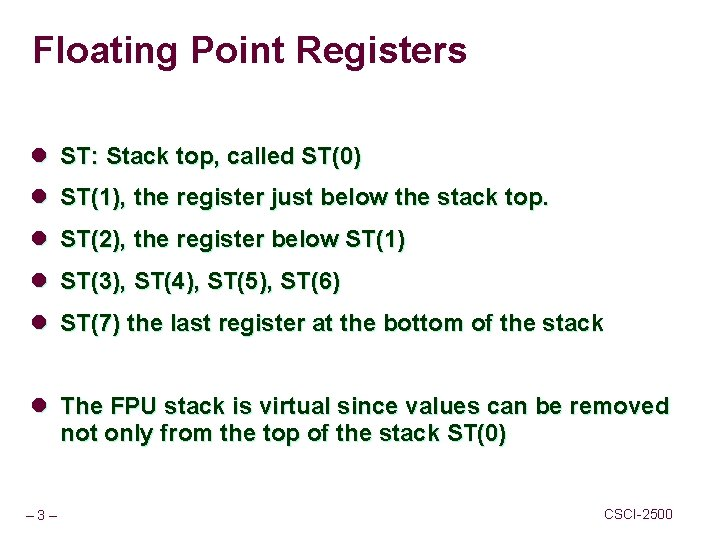Floating Point Registers l ST: Stack top, called ST(0) l ST(1), the register just below the stack top. l ST(2), the register below ST(1) l ST(3), ST(4), ST(5), ST(6) l ST(7) the last register at the bottom of the stack l The FPU stack is virtual since values can be removed not only from the top of the stack ST(0) – 3– CSCI-2500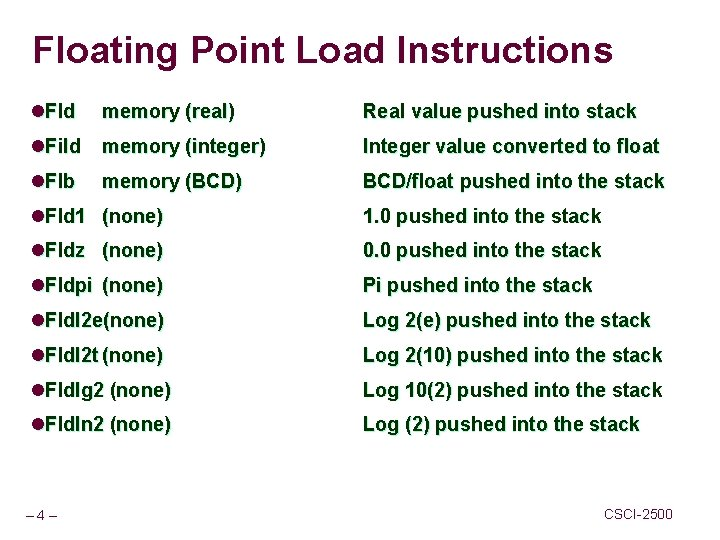Floating Point Load Instructions l. Fld memory (real) Real value pushed into stack l. Fild memory (integer) Integer value converted to float l. Flb BCD/float pushed into the stack memory (BCD) l. Fld 1 (none) 1. 0 pushed into the stack l. Fldz (none) 0. 0 pushed into the stack l. Fldpi (none) Pi pushed into the stack l. Fldl 2 e(none) Log 2(e) pushed into the stack l. Fldl 2 t (none) Log 2(10) pushed into the stack l. Fldlg 2 (none) Log 10(2) pushed into the stack l. Fldln 2 (none) Log (2) pushed into the stack – 4– CSCI-2500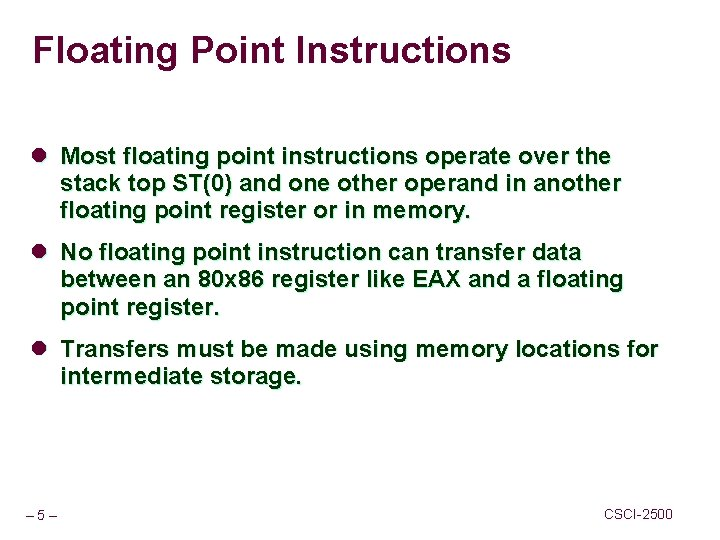Floating Point Instructions l Most floating point instructions operate over the stack top ST(0) and one other operand in another floating point register or in memory. l No floating point instruction can transfer data between an 80 x 86 register like EAX and a floating point register. l Transfers must be made using memory locations for intermediate storage. – 5– CSCI-2500Floating Point Load Examples Consider the following table for the examples fp. Value Real 4 10. 0 int. Value Dword 20 bcd. Value TBYTE 30 – 6– CSCI-2500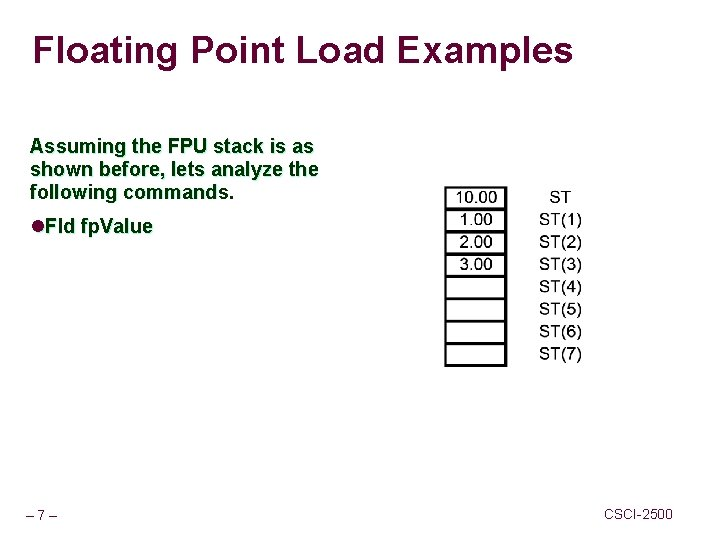Floating Point Load Examples Assuming the FPU stack is as shown before, lets analyze the following commands. l. Fld fp. Value – 7– CSCI-2500Floating Point Load Examples Assuming the FPU stack is as shown before, lets analyze the following commands. l. Fld st(2) l. Notice that the value stored in register ST(2) was pushed again to the top of the stack but was not removed from its current position. – 8– CSCI-2500Floating Point Load Instructions Assuming the FPU stack is as shown before, lets analyze the following commands. l. Fild int. Value l. The integer value is converted to a 10 byte floating point value and pushed into the stack. – 9– CSCI-2500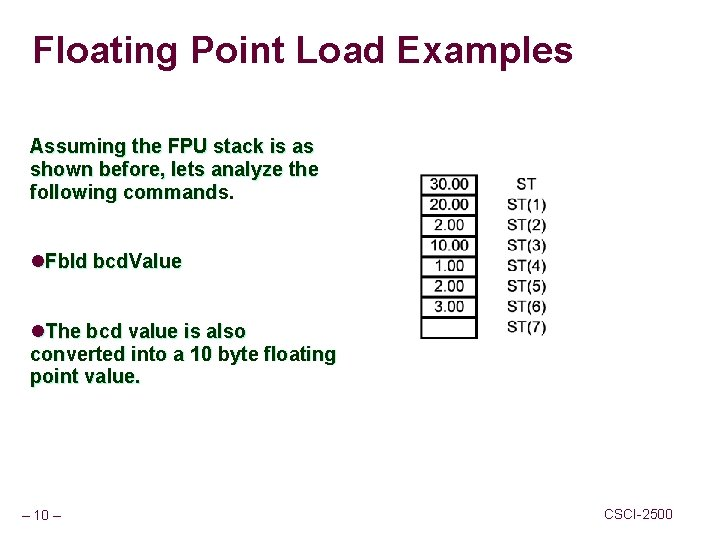Floating Point Load Examples Assuming the FPU stack is as shown before, lets analyze the following commands. l. Fbld bcd. Value l. The bcd value is also converted into a 10 byte floating point value. – 10 – CSCI-2500Floating Point Load Examples Assuming the FPU stack is as shown before, lets analyze the following commands. l. Fldz l. Finit ; initialize the math processor l. Finit is desirable near the beginning of the code since it erases all the FPU registers. – 11 – CSCI-2500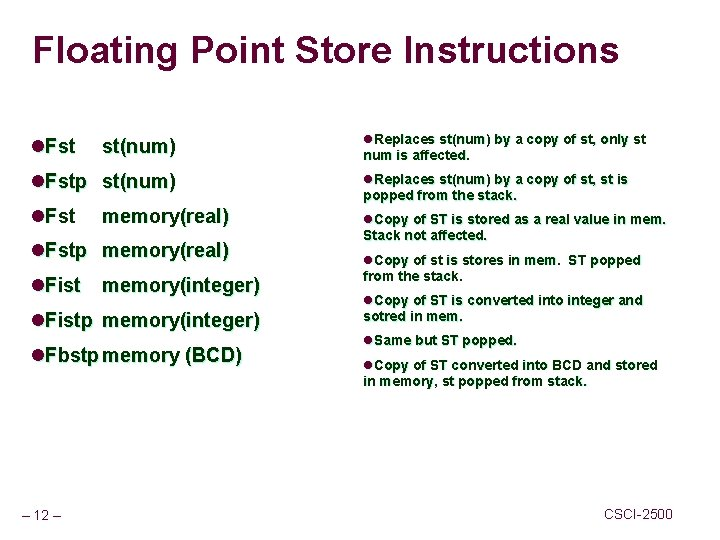Floating Point Store Instructions l. Fst st(num) l. Fstp st(num) l. Fst memory(real) l. Fstp memory(real) l. Fist memory(integer) l. Fistp memory(integer) l. Fbstp memory (BCD) – 12 – l. Replaces st(num) by a copy of st, only st num is affected. l. Replaces st(num) by a copy of st, st is popped from the stack. l. Copy of ST is stored as a real value in mem. Stack not affected. l. Copy of st is stores in mem. ST popped from the stack. l. Copy of ST is converted into integer and sotred in mem. l. Same but ST popped. l. Copy of ST converted into BCD and stored in memory, st popped from stack. CSCI-2500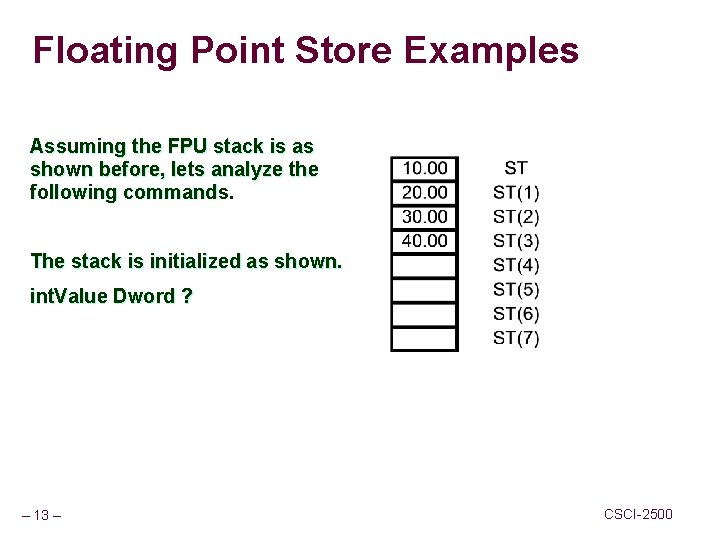Floating Point Store Examples Assuming the FPU stack is as shown before, lets analyze the following commands. The stack is initialized as shown. int. Value Dword ? – 13 – CSCI-2500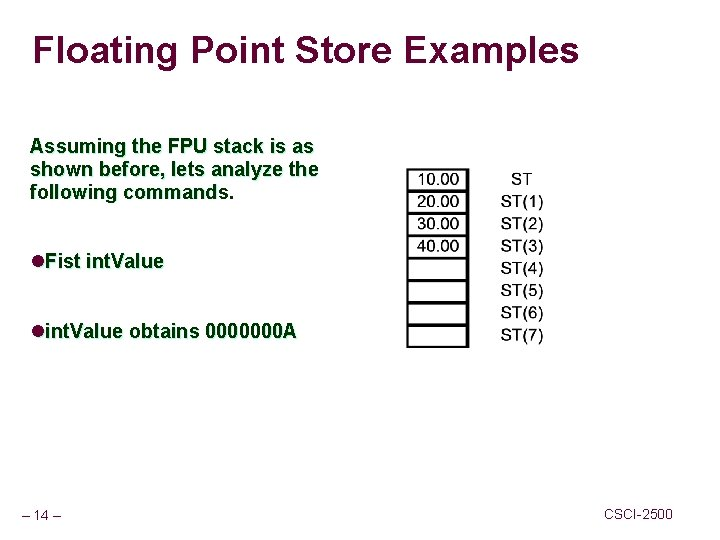Floating Point Store Examples Assuming the FPU stack is as shown before, lets analyze the following commands. l. Fist int. Value lint. Value obtains 0000000 A – 14 – CSCI-2500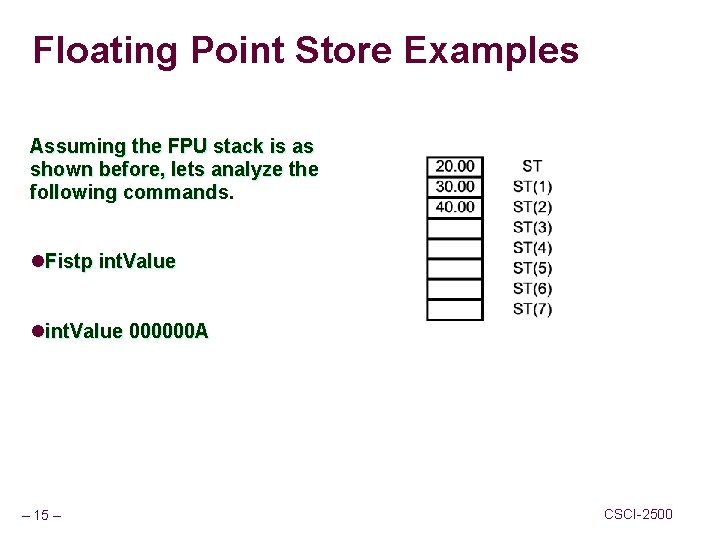Floating Point Store Examples Assuming the FPU stack is as shown before, lets analyze the following commands. l. Fistp int. Value lint. Value 000000 A – 15 – CSCI-2500Floating Point Store Examples Assuming the FPU stack is as shown before, lets analyze the following commands. Original state The situation is more confusing when the destination is another floating point register. l. Fst st(2) l. Fstp st(2) The first command copies the content of the ST into St(2) Fstp st(2) The second does the same and then pops ST from the stack. – 16 – CSCI-2500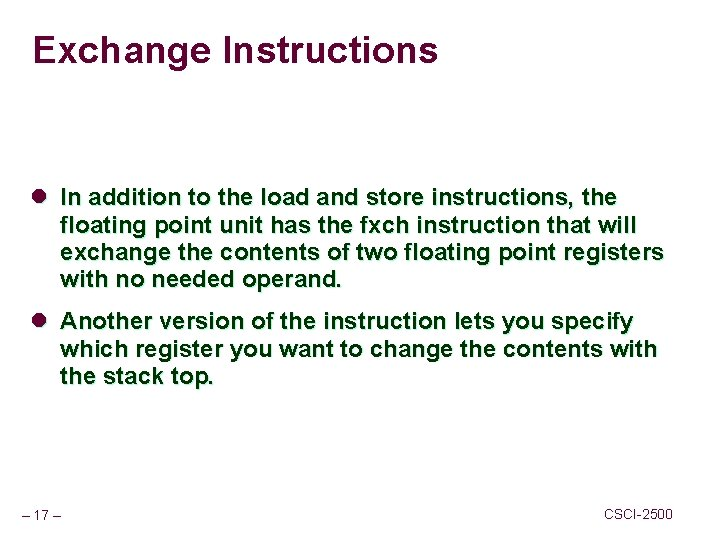Exchange Instructions l In addition to the load and store instructions, the floating point unit has the fxch instruction that will exchange the contents of two floating point registers with no needed operand. l Another version of the instruction lets you specify which register you want to change the contents with the stack top. – 17 – CSCI-2500Exchange Instruction n Fxch ; exchanges ST and ST(1) Fxch st(num) ; exchanges ST and ST(num); n Examples: n l Finit; l Fld 1; l Fldz; l Fxchg; n – 18 – What are the contents of ST and ST(1)? CSCI-2500Floating Point Addition Instructions l The floating point unit contains special instructions for addition purposes. l There are versions of theses instructions to add the contents of ST to another register, the contents of another register to ST, a real number from memory or even an integer number. There are no special instructions for BCD numbers. l The instructions that have a “p” at the end remove the stack top from the floating point registers. – 19 – CSCI-2500Floating Point Addition Examples Assume we have the stack: After executing: Fadd st, st(3) 50. 00 20. 00 30. 00 40. 00 – 21 – ST ST(1) ST(2) ST(3) ST(4) ST(5) ST(6) ST(7) CSCI-2500Floating Point Addition Examples l Using the results from the previous example, assume with run the follogin instructions: n n Fp. Value Real 4 5. 0 int. Value Dword 1 l Fadd fp. Value l Fiadd int. Value n – 22 – What is the result of the floating point stack registers? CSCI-2500Floating Point Addition Examples Original Stack 50. 00 20. 00 30. 00 40. 00 – 23 – ST ST(1) ST(2) ST(3) ST(4) ST(5) ST(6) ST(7) After instructions 56. 00 20. 00 30. 00 40. 00 ST ST(1) ST(2) ST(3) ST(4) ST(5) ST(6) ST(7) CSCI-2500Floating Point Addition Examples Finally after the instruction: Faddp st(2), st 56. 00 20. 00 30. 00 40. 00 – 24 – ST ST(1) ST(2) ST(3) ST(4) ST(5) ST(6) ST(7) 20. 00 86. 00 40. 00 ST ST(1) ST(2) ST(3) ST(4) ST(5) ST(6) ST(7) CSCI-2500Floating Point Substraction Instructions l Substraction instructions are very similary to the corresponding addition instructions. We have 12 of them, divided into two different groups. l The first six are equivalent to the addition instructions. l The second six, are the same except that the operands are the opposite, since substraction is not commutative. – 25 – CSCI-2500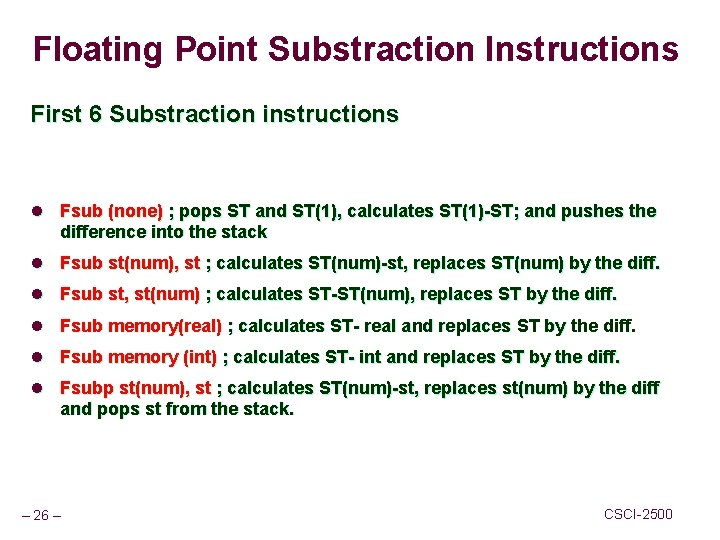Floating Point Substraction Instructions First 6 Substraction instructions l Fsub (none) ; pops ST and ST(1), calculates ST(1)-ST; and pushes the difference into the stack l Fsub st(num), st ; calculates ST(num)-st, replaces ST(num) by the diff. l Fsub st, st(num) ; calculates ST-ST(num), replaces ST by the diff. l Fsub memory(real) ; calculates ST- real and replaces ST by the diff. l Fsub memory (int) ; calculates ST- int and replaces ST by the diff. l Fsubp st(num), st ; calculates ST(num)-st, replaces st(num) by the diff and pops st from the stack. – 26 – CSCI-2500Floating Point Substraction Instructions Substraction instructions “opposite” l Fsubr (none) ; pops ST and ST(1), calculates ST-ST(1), pushes difference onto the stack. l Fsubr st(num), st ; calculates st-st(num), replaces st(num) with the diff. l Fsubr st, st(num) ; calculates st(num)-st, replaces st with the diff. l Fsubr memory(real) ; calculates mem-st, replaces st with the diff. l Fsub rmemory(int) ; calculates mem-st, replaces st with the diff. l Fisubr mem(int) ; calculates mem-st, replaces st with the diff. l Fsubpr st(num), st ; calculates st-st(num), replaces st(num) with the difference and pops st from the stack. – 27 – CSCI-2500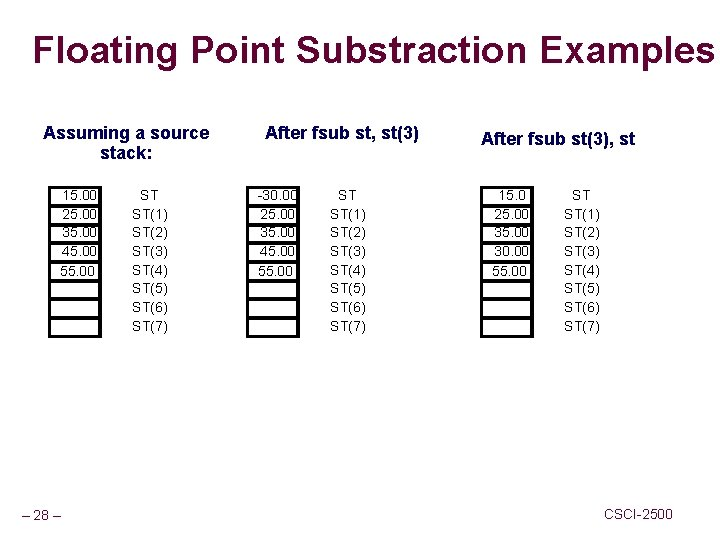Floating Point Substraction Examples Assuming a source stack: 15. 00 25. 00 35. 00 45. 00 55. 00 – 28 – ST ST(1) ST(2) ST(3) ST(4) ST(5) ST(6) ST(7) After fsub st, st(3) -30. 00 25. 00 35. 00 45. 00 55. 00 ST ST(1) ST(2) ST(3) ST(4) ST(5) ST(6) ST(7) After fsub st(3), st 15. 0 25. 00 30. 00 55. 00 ST ST(1) ST(2) ST(3) ST(4) ST(5) ST(6) ST(7) CSCI-2500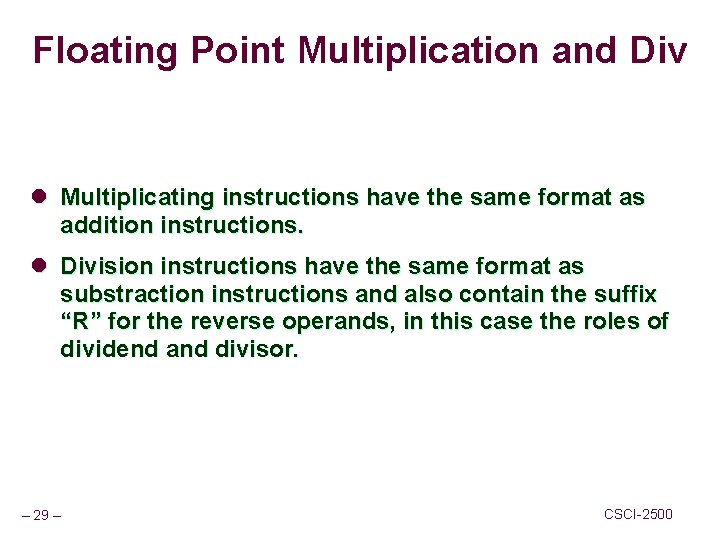Floating Point Multiplication and Div l Multiplicating instructions have the same format as addition instructions. l Division instructions have the same format as substraction instructions and also contain the suffix “R” for the reverse operands, in this case the roles of dividend and divisor. – 29 – CSCI-2500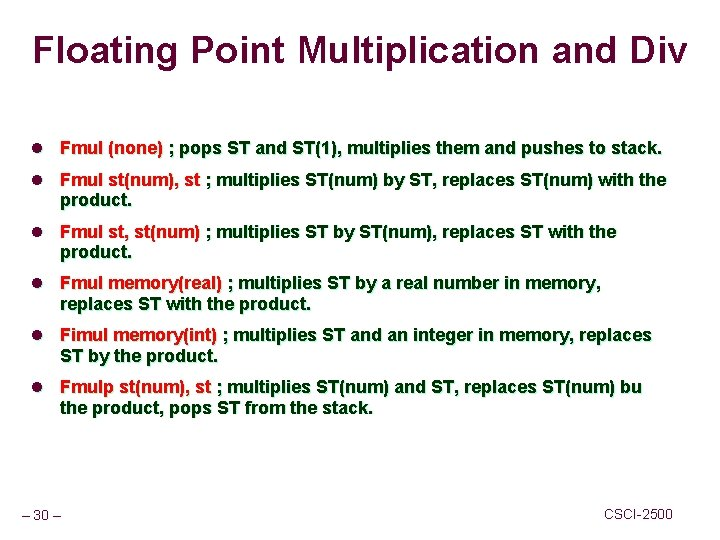Floating Point Multiplication and Div l Fmul (none) ; pops ST and ST(1), multiplies them and pushes to stack. l Fmul st(num), st ; multiplies ST(num) by ST, replaces ST(num) with the product. l Fmul st, st(num) ; multiplies ST by ST(num), replaces ST with the product. l Fmul memory(real) ; multiplies ST by a real number in memory, replaces ST with the product. l Fimul memory(int) ; multiplies ST and an integer in memory, replaces ST by the product. l Fmulp st(num), st ; multiplies ST(num) and ST, replaces ST(num) bu the product, pops ST from the stack. – 30 – CSCI-2500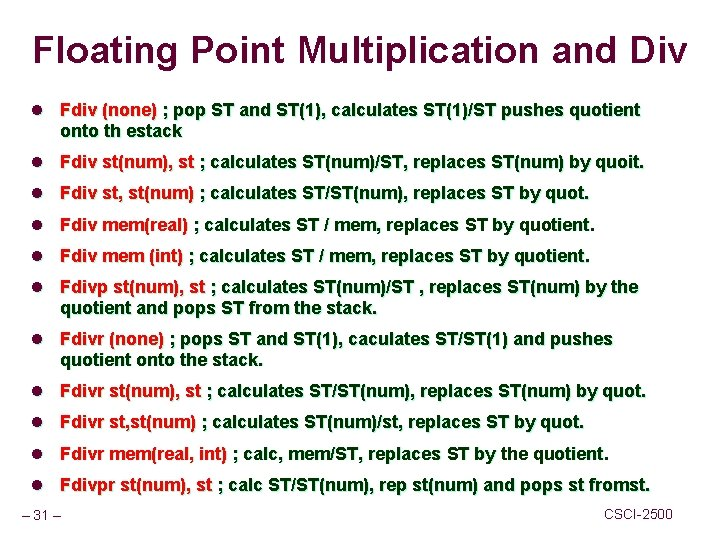Floating Point Multiplication and Div l Fdiv (none) ; pop ST and ST(1), calculates ST(1)/ST pushes quotient onto th estack l Fdiv st(num), st ; calculates ST(num)/ST, replaces ST(num) by quoit. l Fdiv st, st(num) ; calculates ST/ST(num), replaces ST by quot. l Fdiv mem(real) ; calculates ST / mem, replaces ST by quotient. l Fdiv mem (int) ; calculates ST / mem, replaces ST by quotient. l Fdivp st(num), st ; calculates ST(num)/ST , replaces ST(num) by the quotient and pops ST from the stack. l Fdivr (none) ; pops ST and ST(1), caculates ST/ST(1) and pushes quotient onto the stack. l Fdivr st(num), st ; calculates ST/ST(num), replaces ST(num) by quot. l Fdivr st, st(num) ; calculates ST(num)/st, replaces ST by quot. l Fdivr mem(real, int) ; calc, mem/ST, replaces ST by the quotient. l Fdivpr st(num), st ; calc ST/ST(num), rep st(num) and pops st fromst. – 31 – CSCI-2500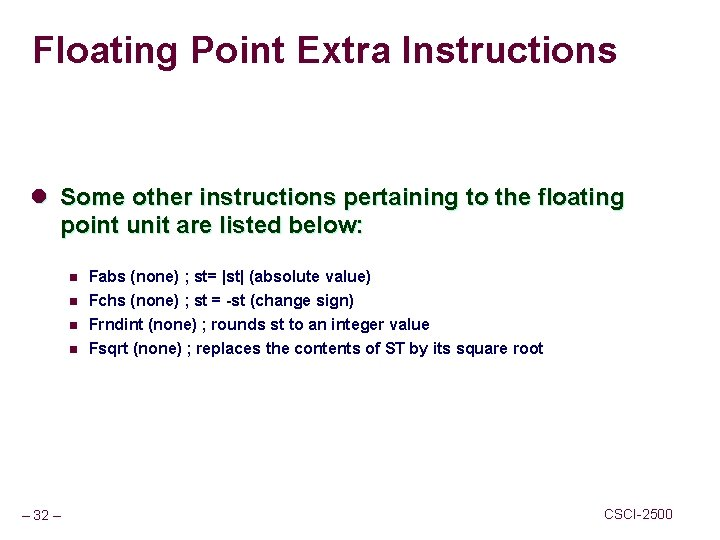Floating Point Extra Instructions l Some other instructions pertaining to the floating point unit are listed below: n n – 32 – Fabs (none) ; st= |st| (absolute value) Fchs (none) ; st = -st (change sign) Frndint (none) ; rounds st to an integer value Fsqrt (none) ; replaces the contents of ST by its square root CSCI-2500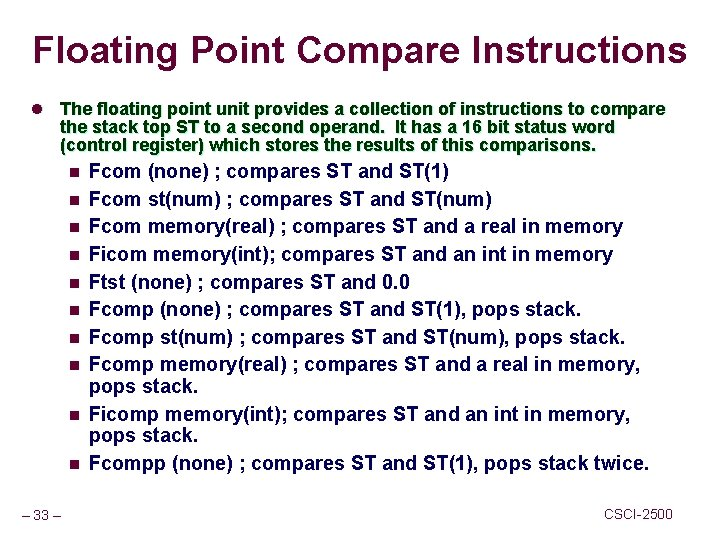Floating Point Compare Instructions l The floating point unit provides a collection of instructions to compare the stack top ST to a second operand. It has a 16 bit status word (control register) which stores the results of this comparisons. n n n n n – 33 – Fcom (none) ; compares ST and ST(1) Fcom st(num) ; compares ST and ST(num) Fcom memory(real) ; compares ST and a real in memory Ficom memory(int); compares ST and an int in memory Ftst (none) ; compares ST and 0. 0 Fcomp (none) ; compares ST and ST(1), pops stack. Fcomp st(num) ; compares ST and ST(num), pops stack. Fcomp memory(real) ; compares ST and a real in memory, pops stack. Ficomp memory(int); compares ST and an int in memory, pops stack. Fcompp (none) ; compares ST and ST(1), pops stack twice. CSCI-2500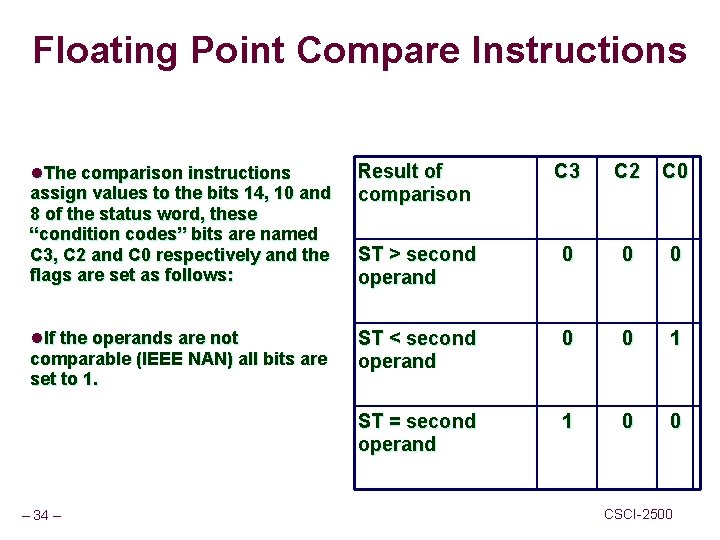Floating Point Compare Instructions l. The comparison instructions assign values to the bits 14, 10 and 8 of the status word, these “condition codes” bits are named C 3, C 2 and C 0 respectively and the flags are set as follows: Result of comparison C 3 C 2 C 0 ST > second operand 0 0 0 l. If the operands are not comparable (IEEE NAN) all bits are set to 1. ST < second operand 0 0 1 ST = second operand 1 0 0 – 34 – CSCI-2500Floating Point Compare Instructions l Status word “conditional codes” cannot be access directly by the conditional jump instructions of the 80 x 86 processor. Special instructions must be used to copy these values to memory locations and to the AX register, they are as follows: n n Fstsw memory word ; copies status register to a memory word. Fstsw AX ; copies status register to AX. Fstcw memory word ; copies control word to memory word. Fldcw memory word ; copies memory word to control word. l Under certain circumstances a cpu might perform more than one FP operation per clock cycle. – 35 – CSCI-2500Floating Point Exercises Solve the following Exercises: Given the following stack • Fld st(2) • Fld fp. Value (value = 10. 0) • Fild int. Value (int = 1) 9. 00 12. 00 23. 00 24. 00 35. 00 Fmul st(3), st Fmul st, st(4) Fmul Fdivr Fchs – 36 – ST ST(1) ST(2) ST(3) ST(4) ST(5) ST(6) ST(7) • Fldpi • Fst st(4) • Fstp st(4) • Fxch st(3) • Fadd st(3), st • Fadd st, st(3) • Faddp st(3), st • Fsub fp. Value (value = 2. 00) • Fisub int. Value (int = 1) • fsqrt CSCI-2500